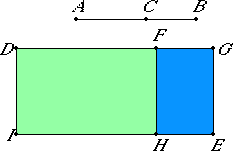# Proposition 78

If from a straight line there is subtracted a straight line which is incommensurable in square with the whole and which with the whole makes the sum of the squares on them medial, twice the rectangle contained by them medial, and further the sum of the squares on them incommensurable with twice the rectangle contained by them, then the remainder is irrational; let it be called that which produces with a medial area a medial whole.
X.35

From the straight line AB let there be subtracted the straight line BC incommensurable in square with AB and fulfilling the given conditions.

I say that the remainder AC is the irrational straight line called that which produces with a medial area a medial whole.II.7

Set out a rational straight line DI. Apply DE, equal to the sum of the squares on AB and BC, to DI producing DG as breadth. Subtract DH equal twice the rectangle AB by BC. Then the remainder FE equals the square on AC, so that AC is the side of FE.

Now, since the sum of the squares on AB and BC is medial and equals DE, therefore DE is medial.

X.22

And it is applied to the rational straight line DI producing DG as breadth, therefore DG is rational and incommensurable in length with DI.

X.22

Again, since twice the rectangle AB by BC is medial and equals DH, therefore DH is medial. And it is applied to the rational straight line DI producing DF as breadth, therefore DF is also rational and incommensurable in length with DI.

Since the sum of the squares on AB and BC is incommensurable with twice the rectangle AB by BC, therefore DE is also incommensurable with DH.

But DE is to DH as DG is to DF, therefore DG is incommensurable with DF.

X.73

And both are rational, therefore GD and DF are rational straight lines commensurable in square only. Therefore FG is an apotome.

X.20

And FH is rational, but the rectangle contained by a rational straight line and an apotome is irrational, and its side is irrational.

And AC is the side of FE, therefore AC is irrational.

Let it be called that which produces with a medial area a medial whole.

Q.E.D.

## Guide

This proposition is used for a few later propositions in Book X starting with X.84.